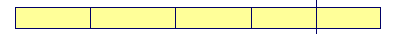S k i l l
i n
A R I T H M E T I C

Lesson 28  Section 2

# Percent increase or decrease

Back to Section 1

Example 1.   A blouse that usually sells for \$32 is on sale at 25% off.  How much do you pay?

Answer.  "25% off" means that a quarter of the price will be subtracted. A quarter of 32 is 8.  (Lesson 15.)  Therefore you will pay \$8 less.  You will pay \$32 − \$8 = \$24.

Example 2.   A VCR that usually sells for \$180 is being sold at a

 discount of 33 13 %.  How much do you pay?
 Answer.  A "discount of 33 13 %" means that a third of the price will be

taken off.  A third of 180 is 60. (Lesson 16, Question 1.)  You will pay

\$180 − \$60 = \$120.

Example 3.   A CD that last week sold for \$15 now costs 10% more.  How much does it cost now?

Answer.  10% of \$15 is \$1.50. (Lesson 4, Question 8.)  Therefore it now costs

\$15 + \$1.50 = \$16.50.

Example 4.   A store increased the price of an item by 25%. By what percent must it decrease the new price in order to restore the original?

Solution.  This problem is best understood in the language of parts. 25% means a quarter.

Let this figure, then, represent the original price divided into quarters.In this figure, it is increased by a quarter.The whole is now in five equal parts: one more than the original. Therefore, to restore the original, it must be reduced by a fifth; which is 20%.  20% -- a fifth -- must be subtracted.

Here is the sequence of names of the parts.

Half, third, fourth, fifth, sixth. And so on.

In any problem of this type, by whichever part the original price was increased, the new price must be reduced by the next part in the sequence.

If the price was increased by a third, then to restore the original, the new price must be reduced by a fourth. If increased by a fourth, it must be reduced by a fifth. And so on.

See Problem 13 .

Example 5  Calculator problem.   An item costs \$39.95, and the tax

 is 8 34 %.  How much will you pay for the item?

Solution.   On simple calculators, press

 3 9 . 9 5 × 8 . 7 5 % +

That is, press the + key.  Do not press = .  See

 43.4456

You will pay \$43.45.

Alternatively, the total cost is 100% of the item plus 8.75%, which is a total of 108.75%.  Press

 3 9 . 9 5 × 1 0 8 . 7 5 %

Example 6.   Calculator problem   Marlene's rent was \$672.50.  It was reduced by 6½%. What is her present rent?

Solution.   This time press − .  Press

 6 7 2 . 5 × 6 . 5 % −

See

 628.788

Her present rent is \$628.79.

Estimations

When using a calculator, and in general, the student should have some idea as to where the answer lies.  With a calculator it is very easy to press a wrong button.

Example 7.   How much is 26.2% of \$44.59?  Choose the best answer:

\$.11    \$1.10     \$11    \$110

Answer.  \$11.  Because 26.2% is close to 25%, which is a quarter.  A quarter of 44 is 11.

Example 8.   How much is 49. 3% of \$205.16?  Choose the best answer:

\$1,020   \$102    \$10.20    \$1.02

Answer.  \$102.   49. 3% is close to 50%, which is half.

Example 9.   98 is approximately what percent of 204?

16%    48%    52%    89%

Answer.   48%.  Because 98 is a little less than half of 204.

Example 10.    What percent of 40 is 11?

6%   24%   27%    9%

Answer.   27%.  Because 11 is a little more than a quarter (25%) of 40, which is 10.

Please "turn" the page and do some Problems.

or

Continue on to the next Lesson.

Section 1 of this Lesson